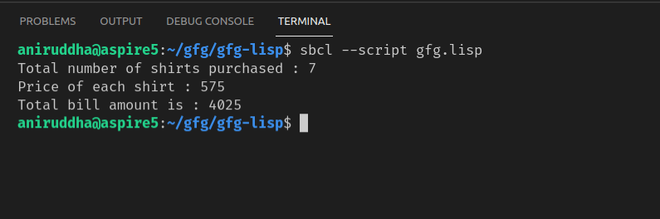Open in App
Not now

# Constants in LISP

• Last Updated : 16 Oct, 2021

In LISP all constants are global variables. Values of constant never change throughout the execution of the program.

### Defining constant in LISP:

New global constants are defined using the DEFCONSTANT construct
Syntax:

```(defconstant name initial-value-form
"documentation-string")```

Example:

Let’s create a global constant whose value will contain the rate of shirt

```(defconstant shirt-rate 575
"shirt-rate is having a constant value of 575")```

Now let’s create a function that will calculate the total bill by taking the number of shirts as input and then multiplying it with our previously defined constant shirt-rate

```(defun calc-total-bill (n)
"Calculates the total bill by multiplying the number of shirts purchased with its constant rate"
(* shirt-rate n))```

Let’s call this function

```(format t "Total number of shirts purchased : 7~%")
(format t "Price of each shirt : ~2d~%" shirt-rate)
(format t "Total bill amount is : ~2d~%" (calc-total-bill 7))```

Code:

## Lisp

 `(defconstant shirt``-``rate ``575``  ``"shirt-rate is having a constant value of 575"``)`` ` `(``defun` `calc``-``total``-``bill (n)``  ``"Calculates the total bill by multiplying the number of``   ``shirts purchased with its constant rate"``  ``(``*` `shirt``-``rate n))`` ` `(``format` `t` `"Total number of shirts purchased : 7~%"``)``(``format` `t` `"Price of each shirt : ~2d~%"` `shirt``-``rate)``(``format` `t` `"Total bill amount is : ~2d~%"` `(calc``-``total``-``bill ``7``))`

Output :We can check if some symbol is the name of a global variable or constant by using boundp predicate, As it is the predicate it returns if it finds the constant with a particular name and will return NIL if it doesn’t.

For example, in our program, we have a constant shirt-rate defined so let’s run this

## Lisp

 `(write (boundp 'shirt``-``rate))``(terpri)``(write (boundp 'rate))`

Output :

```T
NIL```

As expected it has returned T when it finds a constant shirt-rate but returns NIL when it can’t find the constant rate in the program.

My Personal Notes arrow_drop_up